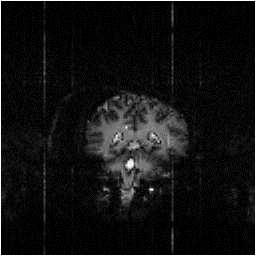### 4.3 Artefact Reduction in fMRI Data

#### 4.3.1 Reduction of Ghost Artefact

One type of artefact which is specific to EPI is the Nyquist, or N/2 ghost. This arises because odd and even echoes are acquired under opposite read gradients, and the data requires reversal prior to image reconstruction. Inaccurate timing of the sampling relative to the switched gradient, temporal asymmetries in the analogue filter or inhomogeneities in the static field cause a modulation of alternate lines in k-space. This leads to a ghost image shifted by N/2 pixels in the phase encode direction. An example of this effect is shown in Figure 4.5a, where the phase of alternate lines in k-space has been altered by a small amount. If the image and ghost overlap, interference occurs leading to fringes in the image as shown in Figure 4.5b.

(a)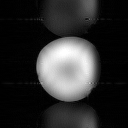(b)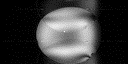##### Figure 4.5.Examples of the effect of the N/2 ghost on phantom images, (a) where ghost and image do not overlap and (b) where ghost and image overlap.

In fMRI, ghosting artefact can cause a number of problems. In the first place, the ghosting can spoil the look of the image, and ghosting of activated regions could lead to apparent 'activation' appearing outside the head. More serious effects occur if the artefact changes with time. Movement of the subjects head causes the interference fringes, of overlapping ghost and image, to change dramatically, with even small displacements having a large effect. Since subject motion is often stimulus correlated, particularly if the experimental paradigm involves movement, the changes of the interference pattern can show up in the statistical analysis as 'activation', as shown in Figure 4.6. It has also been observed on our scanner that the ghosting can change with time as shown in Figure 4.7, from an experiment lasting eight minutes. This probably results from changes in the switched gradient waveform as the coil heats up and its resistance increases.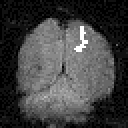##### Figure 4.6. If the interference fringes, caused by the ghost and image overlapping, change with the same period as the stimulus, they appear on the activation images as artefact.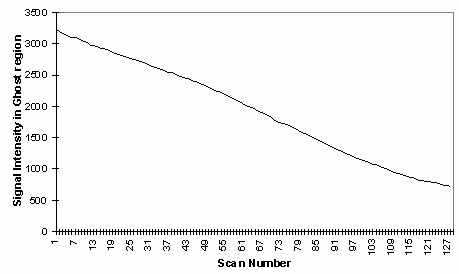##### Figure 4.7. Plot of the signal intensity in a region of the ghost against scan number. Taken from images of a phantom scanned continuously for eight minute.

The N/2 ghost can be reduced by making hardware improvements in filter performance and reducing eddy currents. There is however a limit to the technical improvements that can be made, so a number of techniques for correcting such artefact in post-processing have been proposed. These techniques are described in this section and are tested on phantom and head fMRI data sets to compare their usefulness in this application.

The simplest form of ghost reduction is to apply a phase angle correction to each point in alternate lines of the k-space data. The required phase correction can be determined by eye, and applied to every image in the fMRI data set. The effect of eddy currents can be modelled by an alternate echo phase variation in the read (x-) direction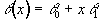(4.2)

so by applying a first order correction, ghosting from such effects can be reduced. A mismatch in the sampling can be corrected by a linear shift of the real and imaginary time data.

All these methods go some way towards removing the N/2 ghost, but will not remove ghosts caused by more complicated phase errors. The subject of N/2 ghosting is extensively covered in a paper by Bruder et. al.  in which a method based on a calibration scan is proposed. Such a scan is acquired with no broadening gradient so, in an ideal case, equivalent points in all the echoes would have the same phase. Bruder's method applies a phase correction to force each echo in the calibration scan to have the same phase, and then applies this same phase correction to the (broadened) image. So if the ith line of the calibration scan has phase qi(x) then the corresponding line in the image is phase corrected by an angle -qi(x) . This can be carried out practically on a time-point by time-point basis, without directly calculating the phase angles, using the formula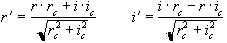(4.3)

where r and i represent the real and imaginary components of the data point and the subscript c represents the calibration scan data.

An alternative form of calibration scan has been proposed by Hu . In this case the reference scan consists of an image acquired under reversed switching gradients. That is to say, if the first line of the normal image is acquired under a positive read gradient, then the first line of the reference image is acquired under a negative read gradient. These two images could be spliced together, so that the final image is made up from only lines that were acquired under a positive read gradient, much in the way that EPI was first proposed . However Hu proposed that the even lines of the reference scan be used to correct the odd lines of the actual image, so that a single reference scan can be used to phase correct a whole set of images. Initially all the lines of the normal images and the reference scan are Fourier transformed in the read direction. Next the even lines of the reference image are reversed. The phase correction q that must be applied to these lines in the corresponding normal image in order for them to have the same phase as the reference image is given by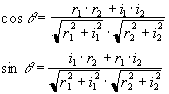(4.4)

where the subscript 1 refers to the normal image and the subscript 2 refers to the reference image. This correction can then be applied to all the images using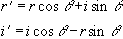(4.5)

again where r is the real component and i is the imaginary component of the complex data point. Finally the images are Fourier transformed along the phase encode direction to form the corrected image.

This technique can be further extended by collecting two reference scans, without the broadening gradient applied, one with a positive read gradient for the first echo and the other with a negative gradient. This has the advantage that there is more signal present in the earlier and later lines of k-space to enable a better calculation of the phase correction required in these lines.

An alternative method that requires no reference scans has been proposed by Buonocore . If two images are constructed, one from only the odd echoes, with the even echoes zero filled prior to Fourier transformation, and one from only the even echoes, with the odd echoes zero filled, then both images will display ghosting, one with a positive ghost, and one with a negative ghost (see Figure 4.8). If there were no phase errors in the odd and even echoes then the phase difference in the object only region (that is where there is no ghost) between these two images would be zero. However if there are phase errors, then a phase difference between equivalent points in the odd and even image of 2q(x,y) can be detected. If the ghost and image do not overlap, it is possible to add the two images together, having applied an appropriate phase correction to one, to form a ghost free image. However if they do overlap then the phase differences are calculated as a function of x only (that is along the read direction) and averaged through all the lines in the object only region as defined by the user. Having determined the phase difference, the original time data can be corrected by Fourier transformation in the read direction, multiplying the odd lines by exp(iq(x)) and the even lines by exp(-iq(x)).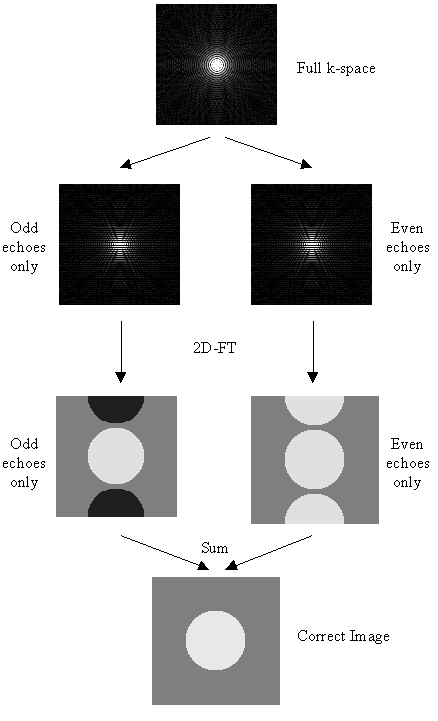##### Figure 4.8. For an image with no ghosting, splitting k-space into even echoes only, or odd echoes only, causes ghosting to appear upon Fourier transformation. The image resulting from the even lines has a positive ghost, whilst the image resulting from the odd lines has a negative ghost. Summing these two image together forms a correct image.

The above five methods were tested on both phantom and head data, with objects that fill and half-fill the field of view. Examples of their ability to correct the ghosting are shown in Figure 4.9a-b. Most of the corrections reduce the ghosting partially in the phantom images. Where the ghost and object overlap, the Hu correction with broadening is slightly better at reducing the ring the edge of the ghost.

To quantify the level of ghosting, regions of interest (ROI) were drawn around the object and the ghost region of the images in which the object half-filled the field of view. Six images of the same phantom, each with different levels of ghosting, were corrected using all five techniques. The mean intensity of the ghost as a percentage of the mean intensity of the object for each technique is shown in Figure 4.9c. The Hu correction without broadening, does not perform as well as might be expected. This is because of the complete failure of the technique in correcting the column in which the external r.f. interference appears, thus producing bright streaks in the ghost region. The Buonocore method appears in both cases to be the best method.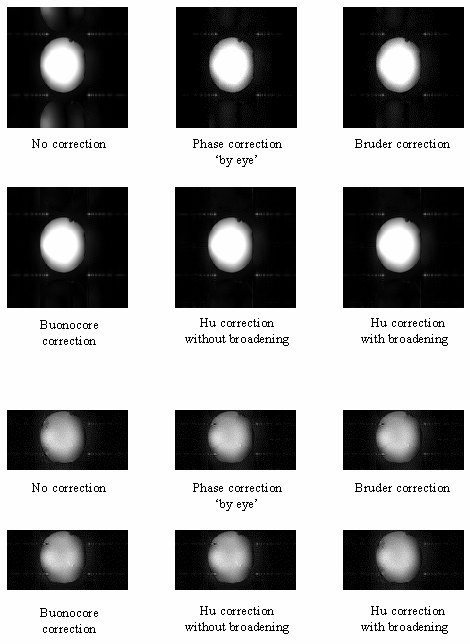##### Figure 4.9a. Examples of the five ghost correction techniques described in the text, applied to 128 x 128 and 128 x 64 matrix size phantom images.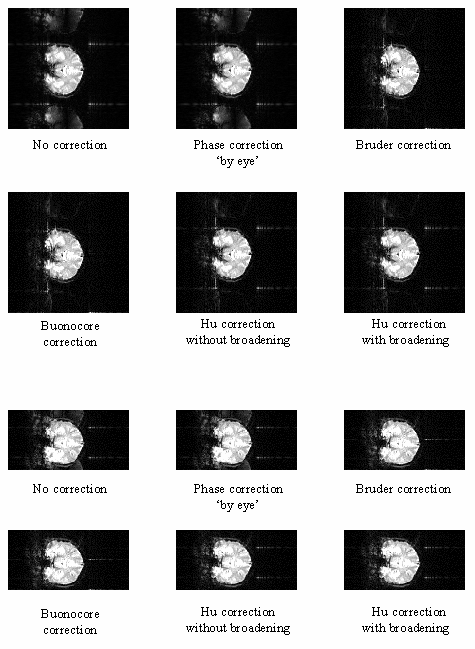##### Figure 4.9b. Examples of the five ghost correction techniques described in the text, applied to 128 x 128 and 128 x 64 matrix size coronal head images.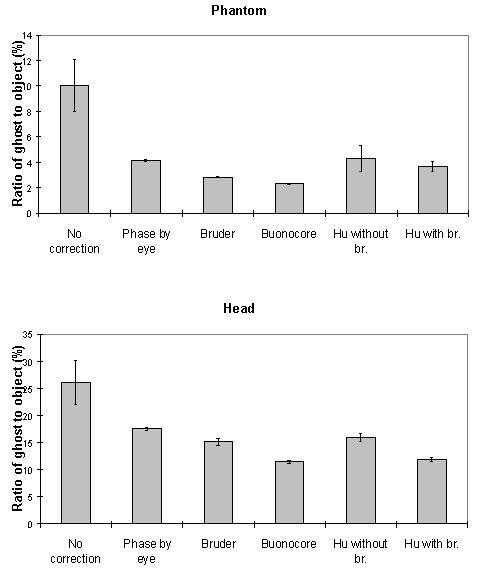##### Figure 4.9c. Ratio of image intensity within the ghost to within the object for the five correction methods explained in the text, based on six images (128 x 128 matrix size) each with slightly different ghosting.

All the above techniques, apart form the Buonocore method, will not correct phase errors that change with time. Since changes in the ghost do occur with time, adaptations of the above techniques are needed if they are to deal with such situations. If ghost only and object only regions can be defined, as is the case if the object does not fill the field of view, then a zeroth or first order alternate line phase correction can be applied which minimises the signal in the ghost region relative to the object region. This technique can work very well in some cases, however if there is any overlap of image and ghost, then the minimisation can occasionally produce obviously incorrect results. Alternatively, the ghost can be minimised by eye on the first image in the set, and then the zeroth and first order phase corrections used to minimise the sum of the square difference between subsequent images and the first. This technique again can generate ridiculous solutions, particularly since this correction has to be carried out before motion correction has been applied. If the assumption is made that the phase errors change linearly with time then a calibration scan can be obtained before and after the fMRI experiment, phase correction calculated, and a linear interpolation of the two angles used to correct intermediate images.

These methods were applied to a test fMRI data set of 64 volume images of a phantom and of a head, acquired over a time of 8 minutes. The first order phase correction method used was one where the signal intensity in the ghost region was minimised by applying the correction shown in equation 4.2. The Bruder correction and the two forms of the Hu correction were applied, such that one correction map was calculated, based on the first image in the set, and the same map applied to all images. Reference scans were also acquired at the end of the experiment in order to produce a correction map for every image, that was a linear interpolation between that for the first and that for the last. The graphs shown in Figure 4.10 show how well each technique corrected the ghost. The striking feature of these plots is that many of the methods, in particular the Hu correction without broadening, made the ghosting worse. This occurs in the columns in the image where the external r.f. interference is at its worse. The effect that this has on the image is shown in Figure 4.11a. Again the Buonocore method works the best in this situation for both head and ghost data. However, this technique does not always work, as is shown in Figure 4.11b. Since this correction is only based on those lines in which the ghost and image do not overlap, the method has failed where the signal intensity in the central rows is low. An appropriate choice of threshold can prevent low signal intensity having an adverse affect on image quality, but this highlights the care that must be used in applying any technique.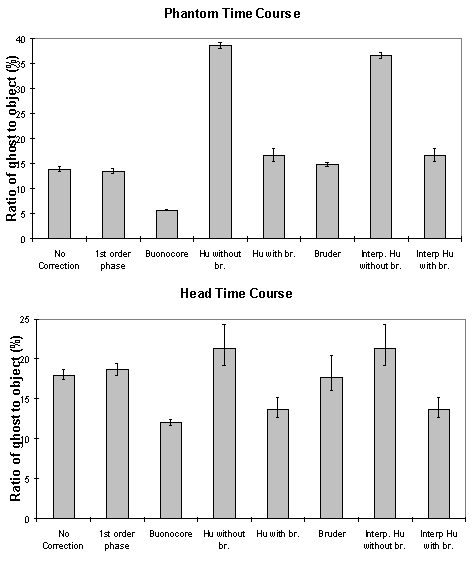##### Figure 4.10. Ratio of image intensity within the ghost to within the object for the five correction methods explained in the text, based on six images (128 x 128 matrix size) each with slightly different ghosting.

(a)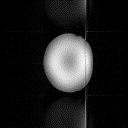(b)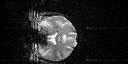##### Figure 4.11. Examples of the failure of two correction methods to remove the N/2 ghosting. (a) Hu correction without broadening, (b) Buonocore correction.

Reducing the ghost artefact is not always easy. A technique that works well on one set of data fails completely on another, and because of this it is useful to be aware of all the possible corrections that could be applied. Since it does not require a reference scan, the Buonocore method would appear to be the most appropriate first choice, however more results obtained from using the method in practice will be necessary to confirm this.

#### 4.3.2 Removal of External RF Interference

As described in section 2.5.3, external r.f. radiation can cause bright dots of artefact to appear in the image. Provided that this interference is at a single discrete frequency, and the sampling is linear, only one or two pixels in the image will be affected and the image can be cleaned up by replacing these pixels by the average of their neighbours. Non-linear sampling however means that the single frequency interference is spread over a wide range of frequencies after Fourier transformation, and consequently appears as a line in the image. Not only does such interference spoil the appearance of the images, fluctuations in its intensity can cause problems with the analysis of the data. In order to cosmetically remove the interference in such a case, it is easier to re-grid the data, such that it appears that it was linearly sampled, and then to remove the few pixels which now contain the interference. The process is illustrated in Figure 4.12.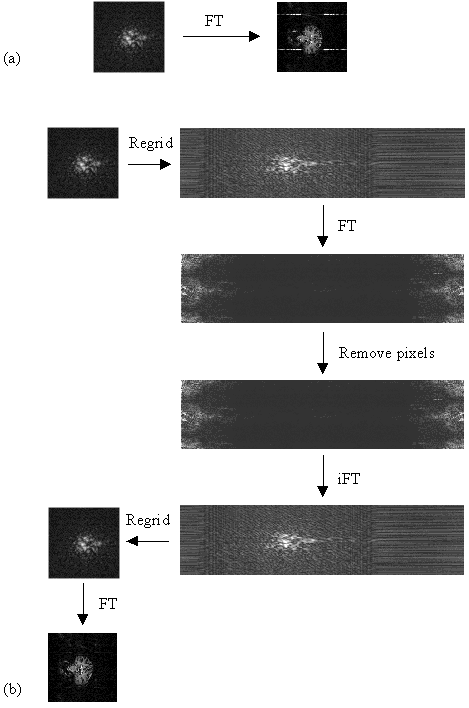##### Figure 4.12. Schematic diagram of process for the removal of r.f. interference bands. (a) Normal image reconstruction using a 2D Fourier transform. (b) The time data is re-gridded to a larger matrix size and Fourier transformed. The interference now appears as a few discrete points on the image and can be removed.

The most effective re-gridding of the time data occurs if each line in the image is expanded to fill a much larger grid, and then the gaps filled in by linear interpolation. The positions in the new grid of length n, of the existing N data points are found by summing under the sinusoidal gradient waveform, and finding the set of points X1...Xk...XN, such that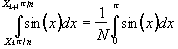.

(4.6)

The values of the intermediate points are obtained by linear interpolation, so that the intensity at the location X in the new grid is given by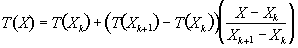.

(4.7)

Re-gridding back from the linear to the sinusoidal grid simply involves extracting the values at the points X1...Xk...XN.

The exact pixels to remove are not easy to determine blindly. It is often the case that they are the brightest pixels, but not always, and because of this software was written so that the user could easily select the pixels that should be removed by looking at the re-gridded image. The application was written in the perl scripting language , with all the data manipulations and calculations carried out in C programs. The user selects the pixels to remove from the expanded image using the mouse.

Examples of data sets before and after cleaning up are shown in Figure 4.13.

(a)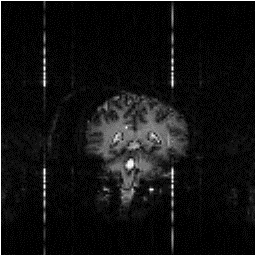(b)